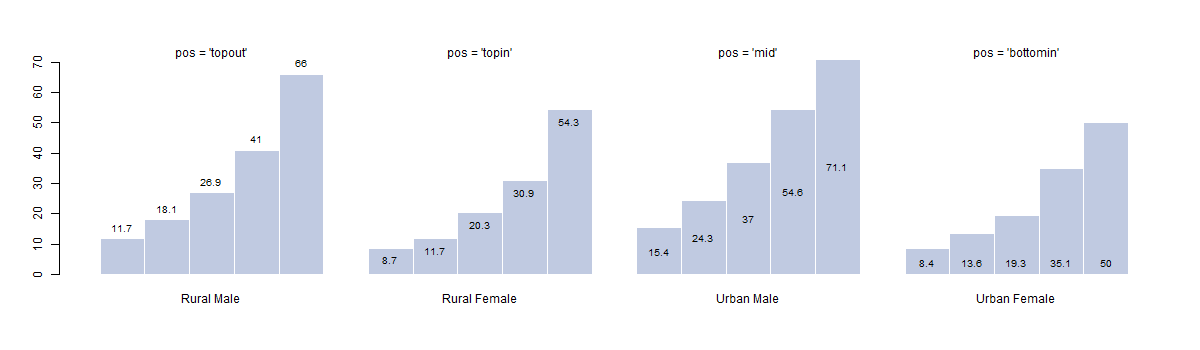BarText {DescTools} R Documentation

## Place Value Labels on a Barplot

### Description

It can sometimes make sense to display data values directly on the bars in a barplot. There are a handful of obvious alternatives for placing the labels, either on top of the bars, right below the upper end, in the middle or at the bottom. Determining the required geometry - although not difficult - is cumbersome and the code is distractingly long within an analysis code. The present function offers a short way to solve the task. It can place text either in the middle of the stacked bars, on top or on the bottom of a barplot (side by side or stacked).

### Usage

BarText(height, b, labels = height, beside = FALSE, horiz = FALSE,
cex = par("cex"), adj = NULL,
pos = c("topout", "topin", "mid", "bottomin", "bottomout"),
offset = 0, ...)


### Arguments

 height either a vector or matrix of values describing the bars which make up the plot exactly as used for creating the barplot. b the returned mid points as returned by b <- barplot(...). labels the labels to be placed on the bars. beside a logical value. If FALSE, the columns of height are portrayed as stacked bars, and if TRUE the columns are portrayed as juxtaposed bars. horiz a logical value. If FALSE, the bars are drawn vertically with the first bar to the left. If TRUE, the bars are drawn horizontally with the first at the bottom. cex numeric character expansion factor; multiplied by par("cex") yields the final character size. NULL and NA are equivalent to 1.0. adj one or two values in [0, 1] which specify the x (and optionally y) adjustment of the labels. On most devices values outside that interval will also work. pos one of "topout", "topin", "mid", "bottomin", "bottomout", defining if the labels should be placed on top of the bars (inside or outside) or at the bottom of the bars (inside or outside). offset a vector indicating how much the bars should be shifted relative to the x axis. ... the dots are passed to the BoxedText.

### Details

The x coordinates of the labels can be found by using barplot() result, if they are to be centered at the top of each bar. BarText() calculates the rest.Notice that when the labels are placed on top of the bars, they may be clipped. This can be avoided by setting xpd=TRUE.

### Value

returns the geometry of the labels invisibly

### Author(s)

Andri Signorell <andri@signorell.net>

BoxedText

### Examples

# simple vector
x <- c(353, 44, 56, 34)
b <- barplot(x)
BarText(x, b, x)

# more complicated
b <- barplot(VADeaths, horiz = FALSE, col=hblue, beside = TRUE)
BarText(VADeaths, b=b, horiz = FALSE, beside = TRUE, cex=0.8)
BarText(VADeaths, b=b, horiz = FALSE, beside = TRUE, cex=0.8, pos="bottomin",
col="white", font=2)

b <- barplot(VADeaths, horiz = TRUE, col=hblue, beside = TRUE)
BarText(VADeaths, b=b, horiz = TRUE, beside = TRUE, cex=0.8)

b <- barplot(VADeaths, horiz = TRUE)
BarText(VADeaths, b=b, horiz = TRUE, col="red", cex=1.5)

# position of the text
old <- par(mfrow=c(3,2), xpd=NA)
off <- c(10, 4, 1, 20, -15)

for(pos in eval(formals(BarText)\$pos)) {
b <- barplot(x, offset=off,
main=gettextf("Textposition pos = '%s'", pos), horiz=TRUE)
abline(h=0)
BarText(x, b, x, offset = off, pos=pos, cex=1.5, horiz=TRUE)
}
par(old)


[Package DescTools version 0.99.51 Index]